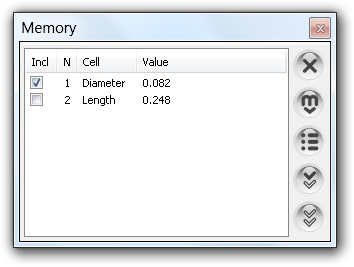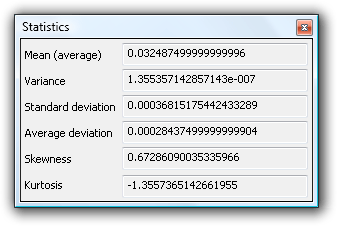The Art of Mathematics

# How to calculate data statistics

## 1. Workflow

Calculating data statistics is straightforward: put dataset into calculator memory and click Statistics.Fig. 1. Statistics is the middle button in Memory window.

Statistics button performs calculation on selected values in Memory or on all values if there is no selection.

## 2. Statistic data

Clicking Statistics button brings in the Statistics window.Fig. 2. Statistics.

Window shows the next values:

ValueDescription
1Mean (average)The sum of elements divided by their number: x = ∑xn / N.
2VarianceVar(x1, ... , xN) = ∑(xnx)2 / (N − 1).
3Standard deviationSquare root of Variance: σ(x1, ... , xN) = √Var.
4Average deviationOr mean absolute deviation: ADev(x1, ... , xN) = ∑|xnx| / N.
5SkewnessOr third moment: Skew(x1, ... , xN) = ∑[(xnx) / σ]3 / N.
6KurtosisOr fourth moment: Kurt(x1, ... , xN) = ∑[(xnx) / σ]4 / N − 3.
Table. 1. Statistic estimators.

## 3. Example

Let us have {a1, a2, a3, a4} data set, put it into calculator memory:

``````2.1−>mem[a1];
2.3−>mem[a2];
2.4−>mem[a3];
3.3−>mem[a4];``````

Fire Ctrl+Shift+= to put the values into memory. Now, click Statistics button in the Memory window to perform calculations — Statistics window is displayed with results.

Now, suppose, we want to exclude a4 value from our data set. Click Invert selection button in the Memory window — all values get selected — and uncheck the a4 value. Click Statistics button again to recalculate statistics this time for truncated set {a1, a2, a3}.